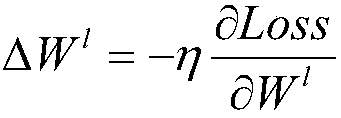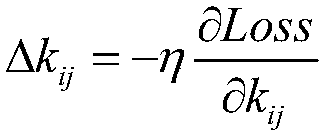# Blower fan blade fault identification method based on deep level characteristic extraction

## A fan blade and feature extraction technology, applied in character and pattern recognition, computer parts, image data processing, etc., can solve the problems of unable to find potential faults of fan blades, unable to judge the type of blade fault, unable to predict faults, etc.

Inactive Publication Date: 2017-09-12
XI AN JIAOTONG UNIV
2 Cites 25 Cited by

## AI-Extracted Technical Summary

### Problems solved by technology

With the continuous expansion of wind power generation scale, the traditional manual inspection can no longer meet the daily inspection needs of wind power generation, and the demand for efficient diagnosis methods for fan blade faults is becoming more and more urgent.
The traditional fault diagnosis method for wind turbine blades is manual inspection, which requires inspectors to climb up the wind turbine and rely on experience to judge the damage of the blad...
View more

## Abstract

The invention discloses a blower fan blade fault identification method based on deep level characteristic extraction. The method comprises steps that an ImageNet image training depth learning neural network is collected to acquire a convolution kernel, a weight and a bias value, the size of a blower fan blade is further adjusted to the size same as an ImageNet image database, a prediction set and a training set are acquired through division, the training set is inputted into the depth learning neural network, and 4096 values of a previous layer of an output layer are extracted and are taken as characteristic values. All the 4096 characteristic values of the training sample are inputted to a support vector machine model for training, 4096 characteristic values of test machine blower fan blade images are further extracted through the depth learning model and are inputted to the trained support vector machine model, and the fault result is acquired. Blower fan blade fault types can be relatively well identified through pictures, management personnel is facilitated to make corresponding processing, and a management level of a wind power plant can be effectively improved.

Application Domain

Image enhancementImage analysis +1

Technology Topic

Image databaseDeep level +10

## Image

•••## Examples

• Experimental program(1)

### Example Embodiment

Hereinafter, referring to FIG. 1, the present invention will be described in more detail with reference to image fault recognition of a fan blade as an example.
The present invention is based on a deep learning fan blade image fault recognition method, and the steps are as follows:
Step 1: Adjust the image size in ImageNet image database to 227*227*3 as the deep learning training set {X i ,Y i },i=1,2...n, where X i Represents the image, its size is 227*227, 3 represents the RGB three-color channel, Y i Indicates the category label to which the image belongs, and its value is between 1 and 1000, a total of 1000 categories;
Step 2: Build a deep learning neural network model that includes 5 layers of convolutional layers, 3 layers of pooling layers, and 2 layers of fully connected layers. Convolution and pooling operations on the training set images include the following steps:
(1) Input the image of the training set size of 227*227*3 into the deep learning neural network C1 convolution layer, pass 96 convolution templates of size 11*11, set the step size to 4, and convolve the training image Operate and use the RELU excitation function to keep the feature value between (0,1) to obtain a feature image with a size of 55*55*96;
(2) Input the feature image obtained in step (1) into the deep learning neural network P1 pooling layer, the pooling template is 3*3, the step size is set to 2, and the feature image with the size of 27*27*96 is obtained;
(3) Input the feature image obtained in step (2) into the deep learning neural network C2 convolutional layer, pass 256 convolution templates with a size of 5*5, set the step size to 1, and use the RELU excitation function to set The feature value is kept between (0,1), and a feature image with a size of 27*27*256 is obtained.
(4) Input the feature image obtained in step (3) into the deep learning neural network P2 pooling layer, the pooling template is 3*3, the step size is set to 2, and the RELU excitation function is used to keep the feature value at ( Between 0 and 1), a feature image with a size of 13*13*256 is obtained;
(5) Input the feature image obtained in step (4) into the deep learning neural network C3 convolutional layer, pass 384 convolution templates with a size of 3*3, set the step size to 1, and use the RELU excitation function to set The feature value is kept between (0,1), and a feature image with a size of 13*13*384 is obtained;
(6) Input the feature image obtained in step (5) into the deep learning neural network C4 convolutional layer, pass 384 convolution templates with a size of 3*3, set the step size to 1, and use the RELU excitation function to set The feature value is kept between (0,1), and a feature image with a size of 13*13*384 is obtained;
(7) Input the feature image obtained in step (6) into the deep learning neural network C5 convolutional layer, pass 256 convolution templates with a size of 3*3, set the step size to 1, and use the RELU excitation function to set The feature value is kept between (0,1), and a feature image with a size of 13*13*256 is obtained.
(8) Input the feature image obtained in step (7) into the deep learning neural network P3 pooling layer, the pooling template is 3*3, the step size is set to 2, and a feature image with a size of 6*6*256 is obtained;
(9) Input the feature image obtained in step (8) into the fully connected layer F1 of the deep learning neural network, and use 4096 neurons to fully connect each input feature image to obtain 4096 feature values;
(10) Input the feature value obtained in step (9) into the fully connected layer F2 of the deep learning neural network, and use 1000 neurons to fully connect each input feature image to obtain 1000 feature values, which belong to 1000 The probability of the category.
Step 3: Use the reverse conduction algorithm to update the weight and bias layer by layer. The specific steps are as follows:
(1) Calculate the loss function of each training sample:
Loss=-logf(x) y
Where f(x) y Indicates the probability value of the correct prediction in the output layer.
(2) Calculate the error sensitivity value of the fully connected output layer:
Where f′(u L ) Represents the derivative of the output activation function of the L-th layer, y n Is the one-hot code of the sample label, f(x n ) Is the probability value that the sample belongs to each category, and is the dot product, that is, each element is multiplied.
(3) Calculate the error sensitivity value of other fully connected layers:
Where W l+1 Represents the weight of the l+1 layer, T represents transpose, δ l+1 Represents the error sensitivity value of the l+1 layer, f′(u l ) Represents the derivative of the output activation function of the first layer;
(4) Calculate the error sensitivity value of the convolutional layer:
Where W l+1 Represents the weight of the l+1 layer, T represents transpose, δ l+1 Represents the error sensitivity value of the l+1 layer, f′(u l ) Represents the derivative of the output activation function of the first layer;
(5) Calculate the error sensitivity value of the pooling layer:
Among them, conv2 represents the discrete convolution calculation, rot180 represents the rotation of the convolution kernel by 180 degrees, and represents the convolution kernel of the l+1 layer.
(6) Calculate the convolution kernel, weight and bias value derivative:
Where u, v represent the coordinates of the convolution kernel, k ij Represents the convolution kernel parameters, W l Represents the weight, b j Indicates bias, X l-1 Represents the output value of layer l-1, which is X l-1 The result of element-wise multiplication with the l-layer convolution kernel;
(7) Update the convolution kernel, weights and bias values ​​through the derivative values ​​calculated in step (6):
Where η is the learning rate;
Step 4: Repeat step 2 to step 3 until the change value of the convolution kernel, weight and bias value is less than 10 -6;
Step 5: Adjust the fan blade image size to 227*227*3, as shown in Figure 2, and divide it into two groups. One group is used as the training set {X train ,Y train }, where Y train 1 represents trailing edge damage, 2 represents cracks, 3 represents peeling, 4 represents scratches, 5 represents normal, and 6 represents paint damage, a total of 6 types, and the other group is used as a test set {X test ,Y test }, where the number of samples in the training set is 54 and the number of samples in the test machine is 18.
Step 6: Use the convolution kernel, weights and bias values ​​finally obtained in step 4 to input the training set {X train ,Y train }, through steps (1) to (9) in step 2 of the deep learning neural network, 4096 eigenvalues ​​are obtained;
Step 7: Put the 4096 feature values ​​of all training samples obtained in step 6 into the support vector machine for training and learning, solve the classification hyperplane, and obtain the support vector machine model;
Step 8: Put the test set {X test ,Y test }The data is input to the deep learning neural network model as in step 6, and 4096 feature values ​​are obtained;
Step 9: Bring the feature values ​​obtained in step 8 into the support vector machine model trained in step 7, and finally get the classification prediction result Y predict , The comparison prediction and test labels are shown in the following table, and the prediction accuracy rate is 100%.
Ytest Ypredict Ytest Ypredict Ytest Ypredict
1 1 6 6 6 6
2 2 3 3 1 1
3 3 4 4 6 6
2 2 2 2 5 5
4 4 1 1 4 4
5 5 5 5 3 3
The described deep learning training model adopts ImageNet image library, the classifier adopts support vector machine, and the running platform is MATLAB2014A.

## PUM## Description & Claims & Application Information

We can also present the details of the Description, Claims and Application information to help users get a comprehensive understanding of the technical details of the patent, such as background art, summary of invention, brief description of drawings, description of embodiments, and other original content. On the other hand, users can also determine the specific scope of protection of the technology through the list of claims; as well as understand the changes in the life cycle of the technology with the presentation of the patent timeline. Login to view more.
Who we serve
• R&D Engineer
• R&D Manager
• IP Professional
Why Eureka